# Polynomial function

(diff) ← Older revision | Latest revision (diff) | Newer revision → (diff)
A generalization of the concept of an entire rational function (see Polynomial). Letbe a unitary module over an associative-commutative ringwith a unit. A mapping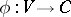is called a polynomial function if, where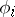is a form of degreeon,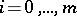(see Multilinear form). Most frequently, polynomial functions are considered whenis a free-module (for example, a vector space over a field) having a finite basis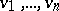. Then the mappingis a polynomial function if and only if, where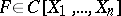is a polynomial overandare the coordinates of an elementin the basis. If hereis an infinite integral domain, the polynomialis defined uniquely.
The polynomial functions on a moduleform an associative-commutative-algebra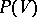with a unit with respect to the natural operations. Ifis a free module with a finite basis over an infinite integral domain, the algebrais canonically isomorphic to the symmetric algebra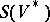of the adjoint (or dual) module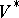, while ifis a finite-dimensional vector space over a field of characteristic 0,is the algebra of symmetric multilinear forms on.